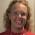Ratio word problem solved with block model and algebra

I guess it is time for some more problem solving, since someone sent this question in.

Two numbers are in the ratio of 1:2. If 7 be added to both, their ratio changes to 3:5. What is the greater number?

We can model the two original numbers with blocks. 1 block and 2 blocks makes the ratio to be 1:2.

|-------|

|-------|-------|

Now add the same thing to both (the 7):
7
|-------|---|

|-------|-------|---|
7
The way I just happened to draw these suggests that I could just split the original block in two, and the problem is solved:
7
|---|---|---|

|---|---|---|---|---|
7
Here, each little block is 7. The original larger blocks are 14 each.

So the original bigger number, which had two larger blocks, is 28, and the smaller number is 14.

Check:
Their ratio is 28:14 = 2:1. If you add 7 to both, you have 35 and 21, and their ratio is 35:21 = 5:3.

Solving the same problem using algebra

The two numbers in the ratio of 1:2 are x and 2x.

Once 7 is added to both, we have x + 7 and 2x + 7. Their ratio is 3:5, and we can write a proportion using fractions:

x + 7 3
------- = ----
2x + 7 5

Cross-multiply to get

5(x + 7) = 3(2x + 7)

5x + 35 = 6x + 21
35 - 21 = x

x = 14

The larger number was 2x or 28. We already checked this earlier.paul.hindess said…
I like these last two problem-solving posts.. Particularly that you accepted your own "good fortune" in the way you first solved this...

Of course, experience shapes our approaches so that we eventually experience "good fortune" more frequently. (-:Anonymous said…
how on earth do you get that? Why do you only add 3 lines and not 7, if you say you add 7 but you only add 3???????

not logic to meThe block method is fine but when numbers get bigger then it might be difficult for elementary students to solve them.Anonymous said…
Thanks. I could set it up algebraically but the block thing eludes me. Perhaps my brain is too old. I got the answer anyway before I did the problem, but my 'intuition' would be no good with huge numbers. I really enjoy your blog.Maria Miller said…
Anonymous,
Those little lines are not units. Imagine the little lines connected together as a solid line. I just drew
several to represent a block, and then a smaller amount to represent 7.Brandan said…
Nice post Honestly never seen the block method for solving the problem before -- only familiar with the algebraic way...rosell clare said…
i'm using block model approach in solving word problems and the children enjoy it...